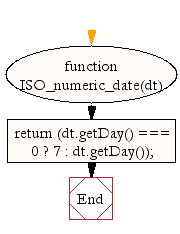# JavaScript: Get ISO-8601 numeric representation of the day of the week

## JavaScript Datetime: Exercise-22 with Solution

Write a JavaScript function to get ISO-8601 numeric representation of the day of the week (1 (for Monday) to 7 (for Sunday)).

Test Data :
dt = new Date();
console.log(ISO_numeric_date(dt));
4
dt = new Date(2015, 10, 1);
console.log(ISO_numeric_date(dt));
7

Sample Solution:-

HTML Code:

``````<!DOCTYPE html>
<html>
<head>
<meta charset="utf-8">
<title>JavaScript function to get ISO-8601 numeric representation of the day of the week (1 (for Monday) to 7 (for Sunday))</title>
</head>
<body>

</body>
</html>
```
```

JavaScript Code:

``````function ISO_numeric_date(dt)
{
return (dt.getDay() === 0 ? 7 : dt.getDay());
}

dt = new Date();
console.log(ISO_numeric_date(dt));

dt = new Date(2015, 10, 1);
console.log(ISO_numeric_date(dt));
```
```

Sample Output:

```3
7
```

Flowchart:Live Demo:

See the Pen JavaScript - Get ISO-8601 numeric representation of the day of the week-date-ex-22 by w3resource (@w3resource) on CodePen.

Improve this sample solution and post your code through Disqus

What is the difficulty level of this exercise?

﻿

## JavaScript: Tips of the Day

Convert tabs to spaces, where each tab corresponds to count spaces

Example:

```const tips_expandTabs = (str, count) => str.replace(/\t/g, ' '.repeat(count));
console.log(tips_expandTabs('\t\tw3resource', 4));
```

Output:

```"        w3resource"
```